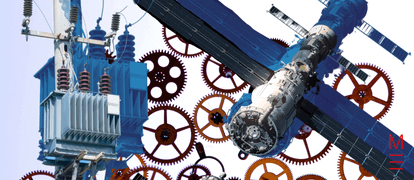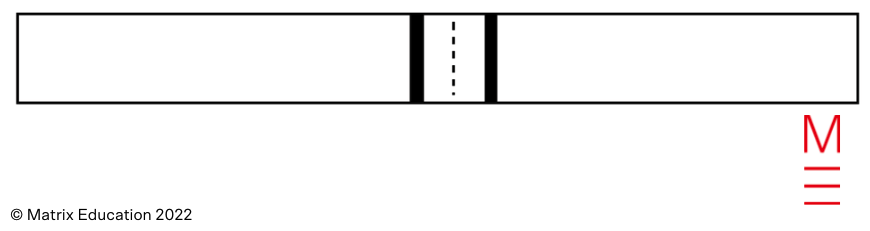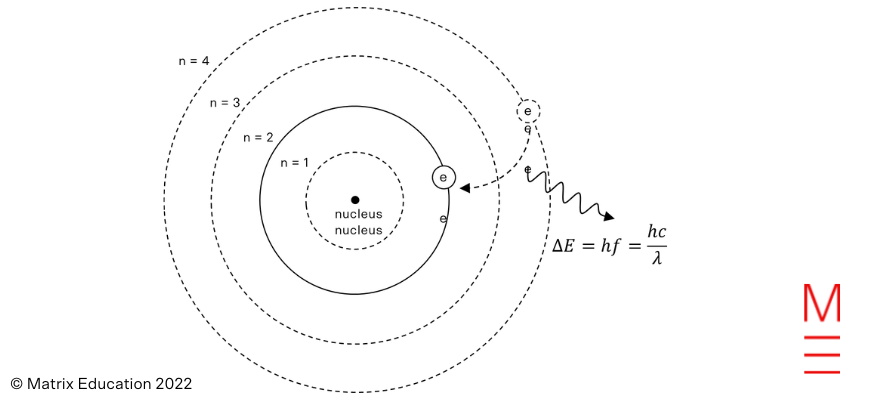# 2022 HSC Physics Exam Paper Solutions

Congratulations on completing your HSC exams! See how you went with our 2022 HSC Physics Exam Paper Solutions.## 2022 HSC Physics Exam Paper Solutions

The 2022 HSC Physics exam was held on 3 November. How did you go? Check your answers with the 2022 HSC Physics Exam Paper Solutions written by the Matrix Science Team!

Find the full 2022 HSC Physics Exam Paper Questions, here.

## Section 1. Multiple Choice

 Question Answer Explanation 1 C This is a step-up transformer. To produce an output voltage 4 times greater than the input, the secondary must have 4 times as many turns. 2 D The hot star emits a continuum of radiation. Atoms and molecules at the surface of the star will absorb specific wavelengths. 3 D The radiation experiences a force towards the positive plate, so it must be a negatively charged particle. 4 C The field lines point from right to left in the space between the magnets. Using the right hand rule, the wire will be pushed into the page. 5 B A neutron has a total charge of zero. 6 A Total energy in an orbit is constant. The planet is travelling fastest at P (Kepler’s Second Law) and has the highest value of kinetic energy at that point. 7 C Using Planck’s equation, $$E=hf$$ and solving for frequency. 8 A The horizontal component of a projectile’s velocity is constant. The vertical velocity decreases in magnitude because of the downwards acceleration due to gravity. 9 C Apply Wien’s Law to solve for temperature. 10 D Square both sides of the equation. $$v_2$$ will be directly proportional to $$\frac{1}{r}$$. 11 B The projectile starts with a positive velocity. It experiences constant negative acceleration, causing it to slow down to zero and eventually have a negative velocity. 12 D The electric field will exert a downwards force on the positive particle, so in order for the net force to be zero (constant velocity) the force from the magnetic field must have been upwards. By the RH rule, this would only occur if the particle was travelling to the right. 13 B Orbital velocity will be the same as it does not depend on mass. Neither does centripetal acceleration. They will also have the same orbital period according to Kepler’s Third Law. However momentum does depend on mass. 14 B Line Y shows a metal with a greater threshold frequency. The photons require a greater energy (and hence frequency) in order to release electrons, which corresponds to a higher work function. 15 A According to Ampere’s Law, the force is proportional to the product of the currents and inversely proportional to the distance between the wires. The two changes cancel each other out. 16 A The binding energy describes the energy that would be required to separate the nucleus into particles. This is higher for He-4. Be-6 is a larger nucleus with more mass. 17 C $$I_y$$ and $$I_z$$ will be equal whenever the vertically polarised filter is at 45 degrees to the vertical 18 D When the droplet is moving downwards at constant velocity, there is no electrical force. The gravitational and frictional forces must balance to give net zero force. When the droplet is moving upwards at constant velocity, there is still a net zero force. The electrical force upwards must balance the combination of gravitational force downwards and the friction opposing the motion. 19 A According to Lenz’s Law, induced currents will always create a magnetic flux that opposes the change which created them, which results in an opposing force. Adding a second lightbulb in series increases the resistance of the circuit. For the same generated EMF, there will be a lower current and hence less opposing force/torque. 20 B Point R is travelling at greatest speed relative to the viewer, as it moves to the right relative to Q. Point Q is travelling at 0.4c relative to the viewer. Point P is stationary relative to the viewer.

## Question 21

a)

X has a greater surface temperature than Y (surface temperature is highest on the left of the diagram).

Y has a greater luminosity than X (luminosity increases upwards on the diagram)

b)

X is performing fusion of hydrogen into helium in the core.

Y is fusing helium atoms into carbon.

## Question 22

The continuous iron core of the transformer is a ferromagnetic material. This ensures the magnetic flux produced by the primary coil is transferred without leakage to the secondary coil. Induced EMF in the secondary is therefore the maximum possible amount.

The laminations of the core prevent large eddy currents from being induced in the transformer core. This improves efficiency by reducing energy dissipation.

## Question 23

This question has multiple possible answers. Two examples are given below.

Cogwheel experiment

A beam of light is passed through the gaps between the cogs of a cogwheel. It reflects off a mirror placed some distance away and returns, passing through the gaps again and on to a screen.

If the wheel is rotated at such a speed that it moves half the distance between the cogs in the time it takes for the light to reflect and return, then the light will strike a cog upon its return and will be blocked from the screen.

The rotation speed of the wheel required to block light from the screen can be used to calculate the time of the light’s journey to and from the mirror. This can be combined with the distance to the mirror, $$d$$, to find the speed of light, $$c = \frac{2d}{t}$$.

Errors would arise from measurements of the cogwheel’s rotation speed, particularly if the beam width was narrower than the cogs, and a range of rotation speeds were able to block the light.

Cavity resonances

A microwave beam of a known frequency can be produced. The beam can be directed to the region between two mirrors, i.e. a cavity, such that it reflects back and forth between them.

The distance between the two mirrors and/or the frequency can be adjusted so as to form a standing wave.

The distance between the mirrors and the order of the standing wave can be determined and used to calculate the wavelength. This can be combined with the known frequency and used to calculate the wavelength, $$c = f\lambda$$.

Errors would arise from measurements of the distance between the mirrors.

## Question 24

a)

The half-life of the substance is 16 hours.

Therefore the decay constant in $$\text{h}^{-1}$$ can be calculated via
$$\lambda = \frac{\text{ln } 2}{t_{1/2}} = 0.043 \text{ h}^{-1}$$

b)

Using $$N = N_0e^{-\lambda t}$$

$$N = 8 \text{ }\mu \text{g}$$
$$N_0 = 80 \text{ }\mu \text{g}$$
$$\lambda = 0.043 \text{ h}^{-1}$$

Solve for $$t$$ in hours: $$t = 53.15 \text{ hours}$$

## Question 25

a)

Calculate the kinetic energy at points 1 and 2 using $$K = \frac{1}{2}mv^2$$

$$K_1 = \frac{1}{2} \times 200 \times 5500^2 = 3.025 \times 10^9 \text{ J}$$
$$K_2 = \frac{1}{2} \times 200 \times 2900^2 = 0.841 \times 10^9 \text{ J}$$

This gives the change in energy $$\Delta K = -2.18 \times 10^9 \text{ J} \approx -2.2 \times 10^9 \text{ J}$$, which has the required magnitude.

b)

We can equate the loss of kinetic energy to an increase in potential energy.

$$\Delta U = U_f – U_i = -\frac{GMm}{r_f} – -\frac{GMm}{r_i}$$

We know the rocket mass and the two positions relative to the planet’s centre.

Solving for the mass of the planet gives $$M = 8.5 \times 10^{23} \text{ kg}$$

## Question 26

a)

The work function $$\phi = 2.9 \text{ eV}$$ equates to an energy of $$4.65 \times 10^{-19} \text{ J}$$ that is lost by the electron as it escapes the metal.

The maximum kinetic energy of the photoelectron is:
$$K = hf – \phi = 6.626 \times 10^{-34} \times 7.5 \times 10^{14} – 4.65 \times 10^{-19} = 3.2 \times 10^{-20} \text{ J}$$

b)

As the electron leaves the metal, it experiences a force downwards due to the electric field (it also experiences a gravitational force but this is so small we can ignore it).

This force will do negative work reducing the kinetic energy of the electron. Once the kinetic energy reduces to zero, the electron will stop. We can solve for the displacement required to achieve this:

$$W = F \times d = qE \times d = 1.602 \times 10^{-19} \times 5.2 \times d = 3.2 \times 10^{-20}$$
$$d = 0.038 \text{ m}$$

## Question 27

a)

Newton considered light to be made of particles called corpuscles. Therefore the expected pattern would be two strips on the screen where the particles passed through the slits.b)

The angles at which the bright lines appear satisfy $$d \sin \theta = m \lambda$$
In this case we are looking at the second order fringe, so $$m=2$$.
$$d = 5.0 \times 10^{-5} \text{ m}$$
$$\lambda = 655 \times 10^{-9} \text{ m}$$
Hence $$\theta = 1.50^\circ$$

c)

Using light with a shorter wavelength causes the angles between bright fringes to become smaller according to $$d \sin \theta = m \lambda$$

The bright lines would appear closer together on the screen.

## Question 28

The energy released in each step depends on the mass defect associated with the reaction. Step Y involves a greater amount of mass converted to energy as calculated here:

Mass of reactants $$= 13.003 + 1.007 = 14.010 \text{ u}$$

Mass of products $$= 14.003 \text{ u}$$

Mass defect $$= 0.007 \text{ u}$$

At a rate of $$931.5 \text{ MeV} / \text{c}^2$$, the energy released in Step Y is $$6.5 \text{ MeV}$$.

## Question 29

We will describe the motion as seen from the ground.

The apple initially has zero vertical velocity. Its horizontal velocity at launch is  the sum of launch velocity to the East and the car’s velocity to the North.

The only force on the apple after it is launched is the gravitational force, acting vertically downwards. There are no forces in the horizontal plane, so the horizontal acceleration is zero and the apple will maintain its horizontal velocity.

The constant vertical acceleration due to gravity causes the velocity to increase downwards at a constant rate until the apple hits the ground.

## Question 30

a)

Einstein postulated that the speed of light should have the same value relative to all observers, regardless of which frame of reference they are in.

The observer inside the train sees the light travel a certain round trip distance from X to Y and back again. The observer outside the train sees the light travel distance as being longer due to the relative horizontal motion of the train.

Both observers agree on the speed of light, so they must disagree on the time interval during which the light was travelling. This is the basis for Einstein’s prediction of time dilation.

b)

The observer inside the train measures the proper time for the journey, which is given as $$15 \text{ ns}$$. Therefore the observer outside will measure a dilated time.

$$t_v = \frac{t_0}{\sqrt{1-v^2/c^2}}$$
$$t_v = 53.6 \text{ ns}$$

## Question 31

Before the Geiger-Marsden experiment, the main model of the atom was Thomson’s “plum pudding” model in which negatively charged electrons were embedded in a solid cloud of positively charged matter that made up the atom.

The Geiger-Marsden experiment involved firing alpha particles at a thin gold foil. Most of the alpha particles passed through the foil without any deflection, but some were deflected, with a few being deflected through very large angles. Rutherford concluded from these results that the positive charge in an atom was concentrated in a very small region at the centre which he referred to as the nucleus. The nucleus exerted repulsive forces on the positively charged alpha particles, and these forces had a greater magnitude the closer the alpha particle passed by.

Rutherford described the electrons as orbiting the nucleus in a ‘planetary’ fashion. However, this model could not account for the fixed wavelengths emitted by hydrogen atoms. Bohr proposed that the electrons orbited the nucleus in quantised orbits at fixed energies. One of his postulates was that electrons could transition between orbits as long as the exact energy difference was absorbed or emitted in the form of a photon with energy $$E = hf$$.Hence the line spectrum of hydrogen corresponded to the allowed electron transitions to lower energies that gave visible light frequencies.

Rydberg’s equation, $$\frac{1}{\lambda} = R_H \left( \frac{1}{n_f^2} – \frac{1}{n_i^2}\right)$$ predicts the wavelengths emitted by hydrogen. The Balmer series, with $$n_f = 2$$, gives the wavelengths shown in the diagram. For example, substituting in $$n_i = 3$$ gives $$\lambda = 656 \text{ nm}$$, corresponding to the rightmost (longest wavelength) line in the spectrum.

## Question 32

a)

The magnets lead to electromagnetic braking. As the conductive metal of the wheel passes between the magnets, eddy currents are induced in the metal due to Faraday’s Law. The induced magnetic flux interacts with the flux of the magnets to oppose the rotation of the flywheel, according to Lenz’s law, resulting in an opposing torque.

b)

The magnets should be moved closer to the outer rim of the flywheel, this will produce a larger opposing torque, $$\tau = Fr$$.
The increased distance of the magnet from the centre of rotation increases $$r$$.

The metal wheel is moving faster near the rim, so the rate of change of flux it experiences will increase, and so will the induced EMF (by Faraday’s Law) and the current. The opposing force will consequently increase.

## Question 33

a)
The projectile’s speed can be found by analysing its motion in a circle.
$$v = \frac{2\pi r}{T} = 20.1 \text{ ms}^{-1}$$

If it is released when the direction is $$45^\circ$$ to the horizontal, we can decompose this total velocity into components. The vertical component of the velocity is then $$20.1 \sin 45^\circ = 14.2 \text{ ms}^{-1}$$ as required.

b)
The projectile’s initial horizontal velocity is $$20.1 \sin 45^\circ = 14.2 \text{ ms}^{-1}$$. To find the horizontal range we must find the time of flight.
From release to landing:
$$s_y = -1.2 \text{ m}$$
$$a_y = -9.8 \text{ms}^{-2}$$
$$u_y = 14.2 \text{ ms}^{-1}$$

Solving $$s = ut + \frac{1}{2}at^2$$ for time and choosing the positive solution gives $$t = 2.98 \text{ s}$$

The horizontal range of the projectile is $$s_x = u_x t = 42.3 \text{ m}$$.

## Question 34

The force exerted on a particle in a magnetic field is $$F = qvB \sin \theta$$. In the case of these particles, they all enter the field perpendicular to the field lines and so we can simplify this to $$F = qvB$$.

Magnetic forces will act as a centripetal force in this situation, as they will exert force with constant magnitude that is always at right angles to the motion of the charged particle. The magnetic force can be equated to the centripetal force $$F_c = \frac{mv^2}{r}$$ in order to derive an expression for the radius of the circular paths:

$$R = \frac{mv}{qB}$$.

The three particles all enter the field with the same speed, and the field is uniform, so all three particles will have the same values of $$v$$ and $$B$$. Any differences between the particle trajectories will therefore be due to differences in their mass to charge ratio, $$m/q$$, and the signs of the charges.

Particle Z is the only one to experience an initial force downwards. The Right Hand rule predicts that it must therefore be a negative charge.

Particle X initially deflects upwards, so by contrast it must be a positive charge. Both X and Z show the same path radius, so we can conclude that they have the same mass-to-charge ratio. Without more information we cannot determine whether their charges have the same magnitude.

Particle Y is also positive according to the Right Hand rule, but its radius of travel is approximately double that of particle X. This means its mass to charge ratio is double that of particle X. Again, without further information we cannot state definitively which of its properties, charge or mass, are different.

## Question 35

If the hypothesis is true, then the force due to gravity between the capsule and the ISS will be equal to the centripetal force $$F_c = \frac{mv^2}{r}$$ required to maintain the path shown.

Gravitational force: $$F_G = \frac{GMm}{r^2} = 8.40 \times 10^{-6} \text{ N}$$

Centripetal force required: $$F_c = \frac{mv^2}{r}= 3.26 \text{ N}$$

The gravitational force between the two objects is not sufficient to provide the necessary centripetal force. Therefore the hypothesis is incorrect and there must be additional forces present to achieve this motion.